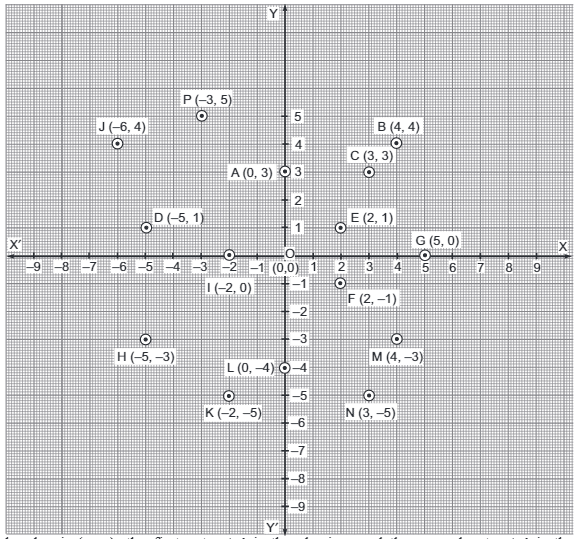Hots Questions - Coordinate Geometry

# Hots Questions - Coordinate Geometry Notes | Study Mathematics (Maths) Class 9 - Class 9

## Document Description: Hots Questions - Coordinate Geometry for Class 9 2022 is part of Coordinate Geometry for Mathematics (Maths) Class 9 preparation. The notes and questions for Hots Questions - Coordinate Geometry have been prepared according to the Class 9 exam syllabus. Information about Hots Questions - Coordinate Geometry covers topics like and Hots Questions - Coordinate Geometry Example, for Class 9 2022 Exam. Find important definitions, questions, notes, meanings, examples, exercises and tests below for Hots Questions - Coordinate Geometry.

Introduction of Hots Questions - Coordinate Geometry in English is available as part of our Mathematics (Maths) Class 9 for Class 9 & Hots Questions - Coordinate Geometry in Hindi for Mathematics (Maths) Class 9 course. Download more important topics related with Coordinate Geometry, notes, lectures and mock test series for Class 9 Exam by signing up for free. Class 9: Hots Questions - Coordinate Geometry Notes | Study Mathematics (Maths) Class 9 - Class 9
 1 Crore+ students have signed up on EduRev. Have you?

Question 1. From the following figure write:
(i) the points whose abscissa is 0.
(ii) the points whose ordinate is 0.
(iii) the points whose abscissa is –5In an ordered pair (x, y), the first entry ‘x’ is the abscissa and the second entry ‘y’ is the ordinate of the point. Let us prepare the following table of ordered pairs of the given points. Also let us write their respective abscissa and ordinate.
From the table we get:
(i) Points with abscissa = 0 are A, L, and O.
(ii) Points with ordinate = 0 are G, I, and O.
(iii) Points with abscissa = –5 are D, H.

 The Point Ordered pair Abscissa Ordinate A (0, 3) 0 3 B (4, 4) 4 4 C (3, 3) 3 3 D (-5, 1) -5 1 E (2, 1) 2 1 F (2, -1) 2 -1 G (5, 0) 5 0 H (-5, -3) -5 -3 I (-2, 0) -2 0 J (-6, 4) -6 4 K (-2, -5) -2 -5 L (0, -4) 0 -4 M (4, -3) 4 -3 N (3, -5) 3 -5 P (-3, -5) -3 5 O (0, 0) 0 0
The document Hots Questions - Coordinate Geometry Notes | Study Mathematics (Maths) Class 9 - Class 9 is a part of the Class 9 Course Mathematics (Maths) Class 9.
All you need of Class 9 at this link: Class 9

## Mathematics (Maths) Class 9

84 videos|352 docs|109 tests
 Use Code STAYHOME200 and get INR 200 additional OFF

## Mathematics (Maths) Class 9

84 videos|352 docs|109 tests

Track your progress, build streaks, highlight & save important lessons and more!

,

,

,

,

,

,

,

,

,

,

,

,

,

,

,

,

,

,

,

,

,

;